# Subtracting Fractions with Like Denominators

Instructor: T.J. Hoogsteen

T.J. is currently a grade 5 teacher and Vice-Principal. He has a master's degree in Educational Administration and is working toward an Ed.D. in Educational Leadership.

Every time you take a part of a whole, you are dealing with fractions. In this lesson, you will learn about subtracting fractions that have like denominators. After solving several examples, you will be a pro!

## Too Much Pizza

The other night I brought home a pizza for dinner. It was a large, 10 piece pizza. I couldn't wait to dig in, and before I knew it I had eaten 5 pieces of pizza. The thing about eating that much is that afterward it is hard to move. You've been there, right? So, as I lay there, I wondered how much of the pizza was left. I could easily figure out how many pieces were left, but I was really wondering about what fraction (equal parts of a whole) was left.

## Subtracting Fractions

Before we get to the good stuff, let's do a quick fraction vocabulary review. In a fraction, there is a number on top that is separated by a line from the number on bottom. The top number is the numerator and it is the number representing how many parts of the whole were taken, or how many parts of the whole are still remaining. The number on the bottom is the denominator. The denominator shows how many equal parts the whole has been divided into.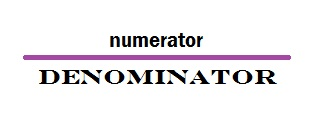OK. Let's get back to our pizza. Before I started eating, there were 10 pieces. If I wrote that as a fraction, it would look like this: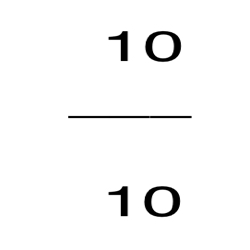Next, I thought about how many pieces I ate, which was 5. So I ate 5 out of 10 pieces. As a fraction I would write it like this: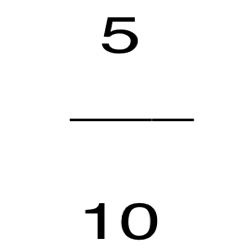Now, to figure out what fraction of the pizza was left, I would take the fraction I started with, and subtract what I ate.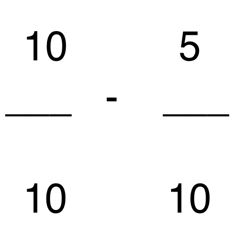But before I subtract, I have to make sure that the denominators in the fractions are the same: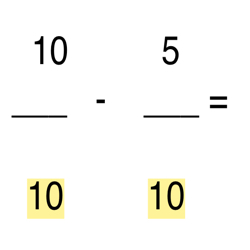Both fractions have the same denominator. Whatever fraction is left over will have the same denominator.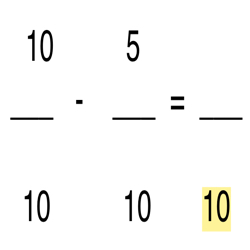Now the next step is to look at the numerators.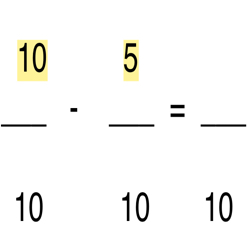As long as the denominators are the same, I just have to subtract the numerators, so I end up with 10 - 5 which equals 5.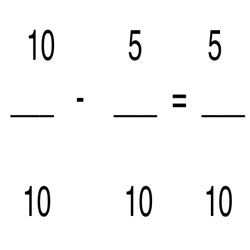To unlock this lesson you must be a Study.com Member.

### Register to view this lesson

Are you a student or a teacher?

#### See for yourself why 30 million people use Study.com

##### Become a Study.com member and start learning now.
Back
What teachers are saying about Study.com

### Earning College Credit

Did you know… We have over 200 college courses that prepare you to earn credit by exam that is accepted by over 1,500 colleges and universities. You can test out of the first two years of college and save thousands off your degree. Anyone can earn credit-by-exam regardless of age or education level.